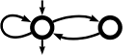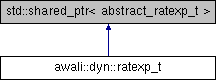Awali Another Weighted Automata library
awali::dyn::ratexp_t Class Reference

Main class for representing rational expresson at the dynamical layer. More...

`#include <ratexp.hh>`

Inheritance diagram for awali::dyn::ratexp_t:## Data Structures

struct  with_int_labels
Helper class that contains convenience factories to build expressions whose labels are integers. More...

struct  with_tuple_labels
Helper class that contains convenience factories to build expressions whose labels are tuples. More...

## Public Member Functions

ratexp_t ()
Buils an automaton_t that is essentially a `nullptr`; should generally not be used. More...

template<class T >
ratexp_t (const std::shared_ptr< T > &ptr, typename std::enable_if< std::is_base_of< abstract_ratexp_t, T >::value, int >::type=0)
Builds a ratexp_t from a shared pointer to a class derived of abstract_ratexp_t; should generally not be used. More...

ratexp_t operator* (ratexp_t exp)

ratexp_t operator+ (ratexp_t exp)
Sum of two rational expressions. More...

## Static Public Member Functions

static ratexp_t from (std::string str, std::string weightset="B", std::string alphabet="auto")
Builds a rational expression from its string representation. More...

static ratexp_t from_context (std::string str, context::labelset_description cd, context::weightset_description, bool fixed_alphabet=true)
Builds the expression represented by `str` and whose labelset is described by `ld` and weightset by `wd`. More...

static ratexp_t from_context (std::string str, context_t ctx, bool fixed_alphabet=true)
Builds a rational expression from a string representation and a context. More...

## Detailed Description

Main class for representing rational expresson at the dynamical layer.

A ratexp_t is essentially a shared pointer to a abstract_ratexp_t. See the documentation of that class for help on the methods on ratexps.

Aside from that, this class contain static methods to constructs rational expressions in different context. See also group Factories.

All examples below assume:

using namespace awali::dyn
Namespace for the dynamical layer of Awali.
Definition: aliases.hh:28
Example (basic)
ratexp_t exp = ratexp_t::from("(a+b)*a(a+b)*"); // Ratexp for words over {a,b} with at least one b.
ratexp_t()
Buils an automaton_t that is essentially a nullptr; should generally not be used.
Definition: ratexp.hh:72
static ratexp_t from(std::string str, std::string weightset="B", std::string alphabet="auto")
Builds a rational expression from its string representation.
ratexp_t exp = ratexp_t::from("(a+b)*a(a+b)*", "B", "abc"); // Same ratexp but the alphabet is {a,b,c} although no 'c' appears in the ratexp.
Example (weights are integers)
ratexp_t exp = ratexp_t::from("(<1>a+<-1>b)*","Z-min-plus"); // Expression that counts the number of a's minus the number of b's
Example (labels are tuples : rational expression for transducers)
ratexp_t exp = ratexp_t::with_tuple_labels::from("([a,a]+[b,b])*",2); // Expression representing the identity over alphabet {a,b}
transducer_t tdc = exp_to_aut(exp);
static ratexp_t from(std::string str, unsigned n, std::string weightset="B")
automaton_t exp_to_aut(ratexp_t ratexp, options_t opts={})
Computes an automaton equivalent to ratexp.
Example (weights are integers, labels are integers)
ratexp_t exp = ratexp_t::with_int_labels::from("(0+1)*1(<2>0+<2>1)*","Z"); // Expression that associated each binary string with its evaluation in base 2.
static ratexp_t from(std::string str, std::string weightset="B")

## ◆ ratexp_t() [1/2]

 awali::dyn::ratexp_t::ratexp_t ( )

Buils an automaton_t that is essentially a `nullptr`; should generally not be used.

## ◆ ratexp_t() [2/2]

template<class T >
 awali::dyn::ratexp_t::ratexp_t ( const std::shared_ptr< T > & ptr, typename std::enable_if< std::is_base_of< abstract_ratexp_t, T >::value, int >::type = `0` )

Builds a ratexp_t from a shared pointer to a class derived of abstract_ratexp_t; should generally not be used.

Both `ptr` and the built automaton_t will share ownership of pointed object.

## ◆ operator*()

 ratexp_t awali::dyn::ratexp_t::operator* ( ratexp_t exp )

## ◆ operator+()

 ratexp_t awali::dyn::ratexp_t::operator+ ( ratexp_t exp )

Sum of two rational expressions.

The documentation for this class was generated from the following file:
• /home/marsault/git-client/vcsn.telecom-paristech.fr/awali.git/awali/dyn/core/ratexp.hh